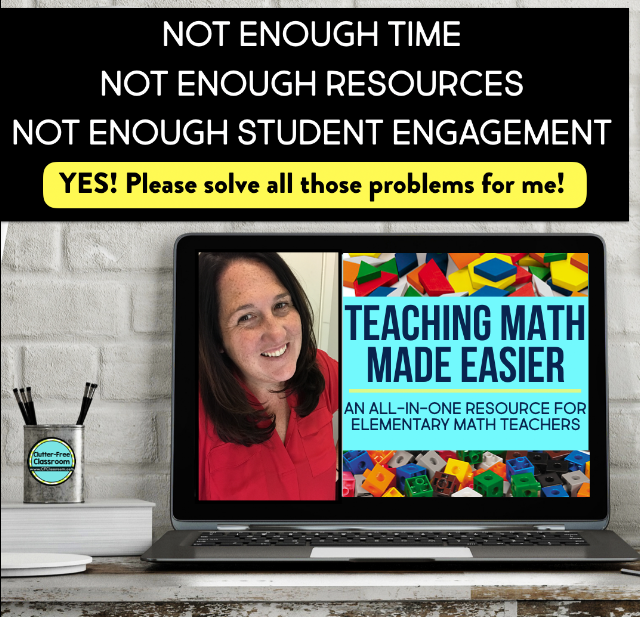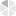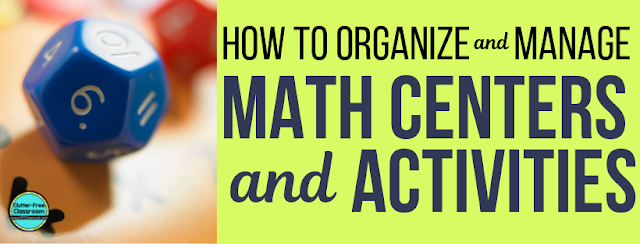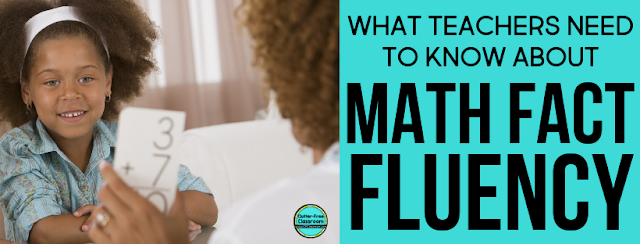# Elementary Math Word Problems

In order for students to become proficient in mathematics, they need to apply their learning to real life situations, which can be achieved through word problems.  During this application, it is essential for students to be exposed to different types of problems and situations. This helps them become flexible thinkers and strong mathematicians.

This experience should not be about following rote procedures and computing correct responses. When solving these types of problems, it is important for students to apply multiple strategies to make sense of the problem and solve it. These experiences should be grounded in strategy application and problem solving, rather than simply computation. Therefore, it is critical to fully equip students with anchor charts, tools, and manipulatives that will motivate and prepare them for these problem solving experiences.

• The different kinds of word problems: Join, separate, part-part-whole, and compare
• Examples of each kind of word problem
• What clue words and phrases are
• The limitations of using clue words and phrases
• Examples of addition and subtraction clue words and phrases
• Common student misconceptions and errors with word problems

TYPES OF WORD PROBLEMS
Read below to learn about the four types of word problems, the subcategories within each of them, and specific examples for all of them.

1. JOIN
This type of word problem involves an action that increases the original amount. There are three kinds: Result unknown, change unknown, and initial quantity unknown.

Result Unknown:
Example: There were 7 kids swimming in the pool. 3 more kids jumped in. How many kids are in the pool now? (7 + 3 = ?)

Change Unknown
Example: There were 8 kids swimming in the pool. More kids jumped in. Now there are 15 kids in the pool. How many kids jumped in? (8 + ? = 15)

Initial Quantity Unknown
Example: There were kids swimming in the pool. 2 kids jumped in. Now there are 6 kids in the pool. How many kids were swimming in the pool at first? (? + 2 = 6)

2. SEPARATE
This type of word problem involves an action that decreases the original amount. There are three kinds: Result unknown, change unknown, and initial quantity unknown.

Result Unknown
Example: There were 12 kids swimming in the pool. 6 of the kids got out of the pool. How many kids are in the pool now? (12 - 6 = ?)

Change Unknown
Example: There were 9 kids swimming in the pool. Some of the kids got out of the pool. Now there are 4 kids in the pool. How many kids got out of the pool? (9 - ? = 4)

Initial Quantity Unknown
Example: There were kids swimming in the pool. 3 of the kids got out of the pool. Now there are 2 kids in the pool. How many kids were in the pool at first? (? - 3 = 2)

3. PART-PART-WHOLE
This type of word problem does not involve an action like the join and separate types. Instead, it is about defining relationships among a whole and two parts. There are two kinds: Result unknown and part unknown.

Result Unknown
Example: There are 5 boys and 9 girls swimming in the pool. How many kids are in the pool? (5 + 9 = ?)

Part Unknown
Example: There are 12 kids swimming in the pool. 8 of them are girls and the rest of them are boys. How many boys are swimming in the pool? (8 + ? = 12)

4. COMPARE
This type of word problem does not involve an action or relationship like the three other types. Instead, it is about comparing two different unrelated items. There are two kinds: Difference unknown and quantity unknown.

Difference Unknown
Example: There are 2 kids in the pool. There are 7 kids in the yard. How many more kids are in the yard than in the pool? (2 + ? = 7 or 7 - 2 = ?)

Quantity Unknown
Example 1: There are 5 kids in the pool. There are 3 fewer kids playing in the yard. How many kids are playing in the yard? (5 - 3 = ?)
Example 2: There are 2 kids in the pool. There are 10 more kids playing in the yard than in the pool. How many kids are playing in the yard? How many kids are playing in the yard? (2 + 10 = ?)

WHAT ARE CLUE WORDS AND PHRASES?
Using clue words and phrases is a strategy to make sense of and solve word problems. It is the idea of training your brain to look for specific words and phrases to determine what operations are needed. Here is an example of this strategy in practice:

Erin reads the problem: Pat has 3 red shirts. He has 2 blue shirts. How many red and blue shirts does he have in all? After reading through the problem once, Erin rereads the problem but this time she is looking specifically for the clue words and phrases she has learned. She highlights or underlines the phrase “in all.” She has learned in class that “in all” signals to the reader that he or she needs to add. This strategy has helped her make sense of the problem (which in this case means that the addition operation is needed), set up an equation (3 + 2 = ?), and solve for the answer (5 shirts).

LIMITATIONS OF USING CLUE WORDS AND PHRASES
It is beneficial for students to be exposed to, directly taught, and given practice with clue words and phrases; however, students need to understand that problems can be solved in many different ways. This is just one tool in their toolkit.  It is not always the most effective strategy to solve a given word problem. For example, students should not be trained to always subtract when they see the word less because they could use a missing addend from addition to solve.  This strategy should be used in tandem with other strategies (e.g. visualization). As students progress through their math education and come across more challenging word problems, this strategy will become less effective. As a result, your students need to be equipped with an abundance of diverse strategies.

KEY VOCABULARY WORDS AND PHRASES IN WORD PROBLEMS
Below is a list of key words and phrases that can be used to solve addition and subtraction word problems.

• Altogether
• Both
• Combine
• In all
• Increase
• Increased by
• Larger
• Larger than
• Longer
• Longer than
• More
• More than
• Perimeter
• Plus
• Sum
• Together
• Total

Subtraction
• Change
• Decreased
• Difference
• Fewer
• Gave away
• How many more
• How many less
• Left
• Left over
• Less
• Less than
• Minus
• Remain
• Shorter than
• Smaller than
• Take away

COMMON MISCONCEPTIONS AND ERRORS
There are common misconceptions and errors that I have seen amongst my students when solving word problems, which include the following:
• Decoding text incorrectly
• Misunderstanding what the problem is asking
• Applying the incorrect operation
• Calculating the addends or the subtrahend from the minuend incorrectly
• Encoding solution in writing incorrectly

What can we do in response to these misconceptions and errors? Try these prompts:
• Can you reread the question aloud to me?
• What is the question asking us to do?
• How can we represent the information and question?
• Can we represent the information and question with an equation?
• What is our first step?
• What is our next step?
• What strategies can we use to help us solve?
• Can you walk me through how you found your answer step by step?
• What do we need to remember when recording our answer?### READ ALL THE BLOG POSTS IN THE ELEMENTARY MATH SERIES:By the way, if you are not already subscribed to The Clutter-Free Classroom newsletter I encourage you sign up. Subscribers receive weekly tips for organizing and managing a classroom as well as exclusive free printables. You can sign up here.